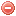# Description

The 10-hour timelag fuel moisture $$MC_{10}$$ is the moisture content of the 10-hour timelag fuels, which consist of dead roundwood 0.25 to 1 inch in diameter and the layer of litter extending from just below the surface to 0.75 inch below the surface (Deeming et al. 1977).

The calculation of the 10-hour timelag fuel moisture model requires the use of fuel sticks. However, an estimation of the 10-hour fuel moisture at midafternoon without the use of fuel sticks is possible according to the formula described here (Bradshaw et al. 1983).

As for the calculation of the 1-hour timelag fuel moisture model, the calculation of the 1-hour timelag fuel moisture model requires daily temperature [°F], relative humidity [%] and fraction of sky cover at early to midafternoon time (Bradshaw et al. 1983).

# Formula

When fuel sticks are not used, the 10-hour fuel moisture $$MC_{10}$$ [%] for midafternoon observation time is estimated in a manner similar to that for the 1-hour fuel moisture model (Cohen & Deeming 1985):

$MC_{10}=1.28\cdot{EMC_{f/a}}$

where $$EMC_{f/a}$$ is the same $$EMC$$ used to calculate the 1-hour timelag dead fuel moisture model.

NB: According to Bradshaw et al. (1983), this model works well for early afternoons in strong continental areas at the approximate latitude of Nebraska (~41°N) in the late summer, but tends to underpredict fuel stick moisture under other conditions.

The 10-hour timelag fuel moisture model is supposed to be calculated on a daily basis. The meteorological data used for its calculation have to be recorded at early to mid-afternoon time (1 to 3 pm).

Deeming et al. (1977)

### Bibliography SearchNo results!

No results were found. Are you sure you searched for a tag?

### Symbols

 Variable Description Unit $$T$$ air temperature °C $$T_{dew}$$ dew point temperature °C $$H$$ air humidity % $$P$$ rainfall mm $$U$$ windspeed m/s $$w$$ days since last rain (or rain above threshold) d $$rr$$ days with consecutive rain d $$\Delta t$$ time increment d $$\Delta{e}$$ vapor pressure deficit kPa $$e_s$$ saturation vapor pressure kPa $$e_a$$ actual vapor pressure kPa $$p_{atm}$$ atmospheric pressure kPa $$PET$$ potential evapotranspiration mm/d $$r$$ soil water reserve mm $$r_s$$ surface water reserve mm $$EMC$$ equilibrium moisture content % $$DF$$ drought factor - $$N$$ daylight hours hr $$D$$ weighted 24-hr average moisture condition hr $$\omega$$ sunset hour angle rad $$\delta$$ solar declination rad $$\varphi$$ latitude rad $$Cc$$ cloud cover Okta $$J$$ day of the year (1..365/366) - $$I$$ heat index - $$R_n$$ net radiation MJ⋅m-2⋅d-1 $$R_a$$ daily extraterrestrial radiation MJ⋅m-2⋅d-1 $$R_s$$ solar radiation MJ⋅m-2⋅d-1 $$R_{so}$$ clear-sky solar radiation MJ⋅m-2⋅d-1 $$R_{ns}$$ net shortwave radiation MJ⋅m-2⋅d-1 $$R_{nl}$$ net longwave radiation MJ⋅m-2⋅d-1 $$\lambda$$ latent heat of vaporization MJ/kg $$z$$ elevation m a.s.l. $$d_r$$ inverse relative distance Earth-Sun - $$\alpha$$ albedo or canopy reflection coefficient - $$\Delta$$ slope of the saturation vapor pressure curve kPa/°C $$Cc$$ cloud cover eights $$ROS$$ rate of spread m/h $$RSF$$ rate of spread factor - $$WF$$ wind factor - $$WRF$$ water reserve factor - $$FH$$ false relative humidity - $$FAF$$ fuel availability factor - $$PC$$ phenological coefficient -

 Suffix Description $$-$$ mean / daily value $$_{max}$$ maximum value $$_{min}$$ minimum value $$_{12}$$ value at 12:00 $$_{13}$$ value at 13:00 $$_{15}$$ value at 15:00 $$_{m}$$ montly value $$_{y}$$ yearly value $$_{f/a}$$ value at fuel-atmosphere interface $$_{dur}$$ duration $$_{soil}$$ value at soil level

 Constant Description $$e$$ Euler's number $$\gamma$$ psychrometric constant $$G_{SC}$$ solar constant $$\sigma$$ Stefan-Bolzmann constant